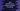# Find the most frequent element in an array using Java## Write a Java program to Find the most frequent element in an array:

Most frequent element means the element that occurs most of the time in an array. To find the most frequent element, follow these steps :

1. We are using a ’map’ to store count of each element in the given array

2. The key of the ’map’ is the number and value is the count of that number

3. First scan the array one by one

4. Check if any key as that particular element exist or not

5. If exist, increment the value of that key by 1.

6. If not, store the value as ’1

7. During the iteration, we are also storing the most frequent value and frequency ( count for that number)

8. These values will update if any value (count for that number) greater than the stored frequency is found.

9. And finally, return the most frequent result found.

``````import java.util.Arrays;
import java.util.HashMap;

public class Main {

public static void main(String[] args) {
int result;

int[] arr1 = new int[]{1, 2, 3, 4, 5, 5, 6, 7, 8, 4, 6, 5};
int[] arr2 = new int[]{1, 2, 3, 4, 5, 6, 7, 8, 9};

result = findFrequentNumber(arr1);
printResult(arr1, result);

System.out.println();

result = findFrequentNumber(arr2);
printResult(arr2, result);

}

/**
* print the result
*
* @param arr    : input array
* @param result : result . if -1 , means all elements frequency is 1
*/
private static void printResult(int[] arr, int result) {
System.out.println(Arrays.toString(arr));
if (result == -1) {
System.out.println("All elements are same ");
} else {
System.out.println(("Most frequent number : " + result));
}
}

/**
* Find most frequent number in a array
*
* @param inputArr : input array
* @return : most frequent element in inputArr
*/
private static int findFrequentNumber(int[] inputArr) {

//create a hashmap to store the count of each element . key is number and value is count for the number
HashMap<Integer, Integer> numberMap = new HashMap<>();

int result = -1; //result will hold the most frequent element for the given array
int frequency = -1; //frequency is the count for the most frequent element

int value;

for (int i = 0; i < inputArr.length; i++) { //scan all elements of the array one by one

value = -1;
// set value as -1
if (numberMap.containsKey(inputArr[i])) {
value = numberMap.get(inputArr[i]); // if the element is in the map, get the count
}
if (value != -1) {
// value is not -1 , that means the element is in the map. Increment the value and check if it is
// greater than the maximum
value += 1;
if (value > frequency) {
//if the value is greater than frequency, it means it is the maximum value
// till now. store it
frequency = value;
result = inputArr[i];
}

numberMap.put(inputArr[i], value); // put the updated value in the map
} else {
//element is not in the map. put it with value or count as 1
numberMap.put(inputArr[i], 1);
}

}

if (frequency == 1)
return -1;

return result;
}

}``````
``````[1, 2, 3, 4, 5, 5, 6, 7, 8, 4, 6, 5]
Most frequent number : 5

[1, 2, 3, 4, 5, 6, 7, 8, 9]
All elements are same
``````